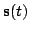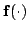Next: Variational Bayesian learning for Up: Missing Values in Hierarchical Previous: Missing Values in Hierarchical

# Introduction

A typical machine learning task is to estimate a probability distribution in the data space that best corresponds to the set of real valued data vectors. This probabilistic model is said to be generative - it can be used to generate data. Instead of finding the distributions directly, one can assume that sourceshave generated the observationsthrough a (possibly) nonlinear mapping:(1)

whereis additive noise. Principal component analysis and independent component analysis are linear examples, but we focus on nonlinear extensions.

It is difficult to visualise the situation if for instance a 10-dimensional source space is mapped to form a nonlinear manifold in a 30-dimensional data space. Therefore, some indirect measures for studying the situation are useful. We use real-world data to make the experiment setting realistic and mark parts of the data to be missing for the purpose of controlled comparison. By varying the configuration of the missing values and then comparing the quality of their reconstructions, we measure different properties of the algorithms.

Generative models handle missing values in an easy and natural way. Whenever a model is found, reconstructions of the missing values are also obtained. Other methods for handling missing data are discussed in . Reconstructions are used here to demonstrate the properties of hierarchical nonlinear factor analysis (HNFA)  by comparing it to nonlinear factor analysis (NFA) , linear factor analysis (FA)  and to the self-organising map (SOM) . Similar experiments using only the latter three methods were presented in .

FA is similar to principal component analysis (PCA) but it has an explicit noise model. It is a basic tool that works well when nonlinear effects are not important. The mappingis linear and the sourceshave a diagonal Gaussian distribution. Large dimensionality is not a problem. The SOM can be presented in terms of (1), although that is not the standard way. The source vectorcontains discrete map coordinates which select the active map unit. The SOM captures nonlinearities and clusters, but has difficulties with data of high intrinsic dimensionality and with generalisation.Next: Variational Bayesian learning for Up: Missing Values in Hierarchical Previous: Missing Values in Hierarchical
Tapani Raiko 2003-07-01